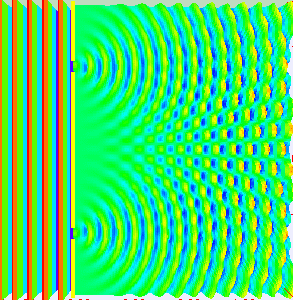# Young's double slit experiment

Crystal037
Homework Statement:
Why parallel beam of light is used for ydse?
Relevant Equations:
I(resultant) = I1+I2+rt(I1*I2cosx)
Where x is the phase difference
I know that ydse is based on interference of light when it passes through an obstacle having almost same aperture as the wavelength of light. Also they should be coherent to have a constant phase difference at every point. But I don't get why the light beams should be parallel?

Homework Helper
Gold Member
2022 Award
Homework Statement:: Why parallel beam of light is used for ydse?
Homework Equations:: I(resultant) = I1+I2+rt(I1*I2cosx)
Where x is the phase difference

I know that ydse is based on interference of light when it passes through an obstacle having almost same aperture as the wavelength of light. Also they should be coherent to have a constant phase difference at every point. But I don't get why the light beams should be parallel?

Do you mean why are the slits are aligned perpendicular to the wavefront? If not, then what do you suggest?

Crystal037
No I mean why we take parallel beam of light i.e. Why do take the light source at the focus of convex lens and then that light is passed through the slit. What if we don't use the parallel light beams.

Homework Helper
Gold Member
2022 Award
No I mean why we take parallel beam of light i.e. Why do take the light source at the focus of convex lens and then that light is passed through the slit. What if we don't use the parallel light beams.

What's a "parallel" beam of light? Parallel to what?

The beam has to be focused at the slits. If not, it misses the slits and you don't have an experiment.

Crystal037
Parallel to each other I am talking about parallel beams of light that pass through both the slit

Homework Helper
Gold Member
2022 Award
Parallel to each other I am talking about parallel beams of light that pass through both the slit
If you have two beams of light that are not parallel to each other, then where are they coming from and what are they aimed at?

If you are proposing an alternative set-up why don't you describe it?

Homework Helper
Basically you want plane waves for a simple setup (what you call parallel beam) in order to have the simplest mathematical description.but the interference will also occur for a screen at an angle, a spherical wavefront coming from the left, etc.
Best thing to do (if you have a chance ) is to do the experiment and play with the setup !

•PeroK